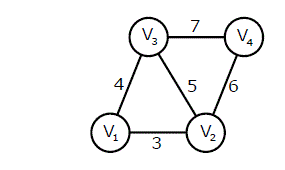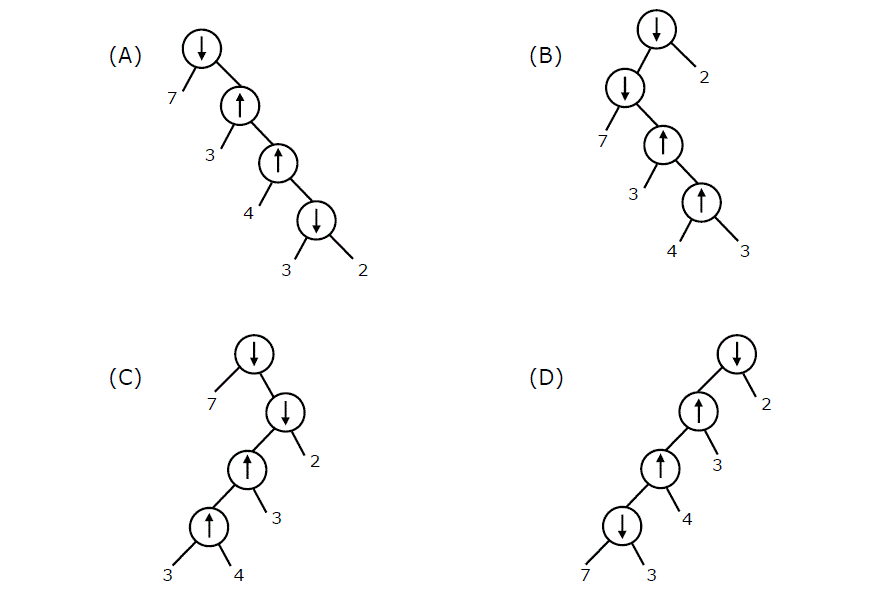# Data Structures and Algorithms | Set 27

• Difficulty Level : Medium
• Last Updated : 11 Nov, 2021

Following questions have been asked in GATE CS 2011 exam.

1) An undirected graph G(V, E) contains n ( n > 2 ) nodes named v1 , v2 ,….vn. Two nodes vi , vj are connected if and only if 0 < |i – j| <= 2. Each edge (vi, vj ) is assigned a weight i + j. A sample graph with n = 4 is shown below.

Attention reader! Don’t stop learning now.  Practice GATE exam well before the actual exam with the subject-wise and overall quizzes available in GATE Test Series Course.

Learn all GATE CS concepts with Free Live Classes on our youtube channel.What will be the cost of the minimum spanning tree (MST) of such a graph with n nodes?
(A) 1/12(11n^2 – 5n)
(B) n^2 – n + 1
(C) 6n – 11
(D) 2n + 1

Minimum spanning tree for 2 nodes would be

``` (v1) _ (v2)
```

Total weight 3

Minimum spanning tree for 3 nodes would be

``` (v1) _ (v2)
|
(v3)
```

Total weight= 3 + 4 = 7

Minimum spanning tree for 4 nodes would be

``` (v1) _ (v2) _ (v4)
|
(v3)
```

Total weight= 3 + 4 + 6 = 13

Minimum spanning tree for 5 nodes would be

``` (v1) _ (v2) _ (v4)
|
(v3)
|
(v5)
```

Total weight= 3 + 4 + 6 + 8 = 21

Minimum spanning tree for 6 nodes would be

``` (v1) _ (v2) _ (v4) _ (v6)
|
(v3)
|
(v5)
```

Total weight= 3 + 4 + 6 + 8 + 10 = 31

We can observe from above examples that when we add kth node, the weight of spanning tree increases by 2k-2. Let T(n) be the weight of minimum spanning tree. T(n) can be written as

T(n) = T(n-1) + (2n-2) for n > 2
T(1) = 0, T(2) = 0 and T(2) = 3

The recurrence can be written as sum of series (2n – 2) + (2n-4) + (2n-6) + (2n-8) + …. 3 and solution of this recurrence is n^2 – n + 1.

2) The length of the path from v5 to v6 in the MST of previous question with n = 10 is

(A) 11
(B) 25
(C) 31
(D) 41

Any MST which has more than 5 nodes will have the same distance between v5 and v6 as the basic structure of all MSTs (with more than 5 nodes) would be following.

``` (v1) _ (v2) _ (v4) _  (v6) _ .  . (more even numbered nodes)
|
(v3)
|
(v5)
|
.
.
(more odd numbered nodes)
```

Distance between v5 and v6 = 3 + 4 + 6 + 8 + 10 = 31

3) Consider two binary operators ‘↑ ‘ and ‘↓’ with the precedence of operator ↓ being lower than that of the ↑ operator. Operator ↑ is right associative while operator ↓ is left associative. Which one of the following represents the parse tree for expression (7 ↓ 3 ­↑ 4 ­↑ 3 ↓ 2)?